# Научный форум dxdy

Математика, Физика, Computer Science, Machine Learning, LaTeX, Механика и Техника, Химия,
Биология и Медицина, Экономика и Финансовая Математика, Гуманитарные науки

 Печатать страницу | Печатать всю тему Пред. тема | След. тема

 Риччи Тензор24/07/12
25
 Всем привет!Я только начал изучать Теорию Относительности. Я имею предстваление о Риеманновском Тензоре, но вот что-то немогу догнать Риччи Тензор. Что он конкретно показывает?Риеманн Тензор можно считать как оператор который берёт 4 вектора и выдает кривизну, которая создана дифицитном углом. Так?Спасибо!Re: Риччи Тензор10/02/11
6786
 Последний раз редактировалось Oleg Zubelevich 24.07.2012, 20:05, всего редактировалось 1 раз. you may speak EnglishRe: Риччи Тензор24/07/12
25
 Oh, Thanks for this opportunity! It's much easier! So, my question relates to the geometrical and physical meaning of the Ricci Tensor. Anyone?Re: Риччи Тензор07/06/11
1890
 GRstudy в сообщении #598782 писал(а):So, my question relates to the geometrical and physical meaning of the Ricci Tensor. Anyone?Well, why you don't try wikipedia? It's messy, but better, than noting.And wikipedia say that this tensor show how volume of manifold different from volume of flat mainfold.And, buy the way, do you try to derive formula for Ricci tesor's component's?Well, I tried, and I noticed some thing, may be it help you to understand geometrical meaning of Ricci tensor, but personally I think it have not obvious geometrical meaning.So, if you take vector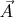$\vec A$ from tangent space to manifold in point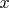$x$ -$TM_x$, and then act on them by commutators$[\partial_\mu, \partial_\nu] = \partial_\mu \partial_\nu - \partial_\nu \partial_\mu$, wher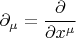$\partial_\mu=\cfrac{\partial}{\partial x^\mu}$ - are usual derivatives, and use, that basis vectors for tangent bundle also changing from point to point according to the law$\partial_\mu \vec e_\nu = \Gamma_{\mu \nu}^\alpha \vec e_\alpha$, where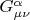$G_{\mu\nu}^\alpha$ - is Christofell simbols. So, if you do all of this you find out that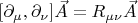$[\partial_\mu,\partial_\nu]\vec A = R_{\mu\nu} \vec A$, where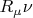$R_\mu\nu$ - component's of Ricci tensor.So, than you may coos coordinate, so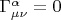$\Gamma_{\mu\nu}^\alpha =0$ in some point, than components of Ricci tensor will be look like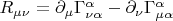$R_{\mu\nu} = \partial_\mu \Gamma_{\nu \alpha}^\alpha - \partial_\nu \Gamma_{\mu \alpha}^\alpha$, where derivatives taken in same point. And hence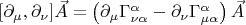$[\partial_\mu,\partial_\nu]\vec A = \left( \partial_\mu \Gamma_{\nu \alpha}^\alpha - \partial_\nu \Gamma_{\mu \alpha}^\alpha \right) \vec A$ which means that if we drug some vector alone a close curve, than when we came back to the start point, resulting vector will save direction, but change lengths. So Ricci tensor show how exactly lengths of vector will be changing, depending of how we have moving it.P.S. I'm also kind of new in GR and differential geometry, so do NOT trust me. I could be wrong.Re: Риччи Тензор
 Заслуженный участник30/01/06
02/09/19
70490
 Последний раз редактировалось Munin 24.07.2012, 21:46, всего редактировалось 1 раз. GRstudy в сообщении #598773 писал(а):Риеманн Тензор можно считать как оператор который берёт 4 вектора и выдает кривизну, которая создана дифицитном углом. Так?Riemann tensor is hardly that simple. I think of it as of an operator, which takes 2 vectors, considers a plane stretched on them, and a travel along a small contour in that plane; and then after such travel the initial basis, carried along the contour, will undergo some linear transform, for example a spatial turn (actually, exactly a spatial turn); and this linear transform can be descibed by a matrix of coefficients linkin initial and final basis. Which makes the tensor taking another two vectors, to yield a coefficient between them.Only in a case when both first and second pair of vectors lie in the same plane, it makes such a transform show an angular defect, because a vector in a fixed direction towards a line of the contour (e. g. triangular or circular) will be found after the travel turned inside or outside by some angle. Thus, the angular deficit seems to me insufficient to show all the details shown by the Riemann tensor. I can see no way a component like$R^1{}_{234}$ (with four orthogonal vectors) could be expressed by the means of some angular defects of some planes, though maybe I'm mistaken.For the meaning of a Ricci tensor, maybe this link http://en.wikipedia.org/wiki/Ricci_curvature#Properties can be of some help.-- 24.07.2012 22:46:53 --By the way, the best explanations of geometry applied in GR can be found in the Gravitation, by Misner, Thorne, Wheeler, easily downloaded.Re: Риччи Тензор24/07/12
25
 Thanks for replies!It's not exactly what I was asking for. At this stage, I am not interested in mathematical viewpoint of Riemann or Ricci tensor. I have followed Leonard Susskind's lectures on General Relativity on YouTube so I have some mathematical insight into this. Apart from mathematics, what is intuitive way of describing these two tensors? Riemann tensor is obviously related to curvature. On a surface of a curved space, if we do parallel transport of a vector around a closed loop, is there is curvature, the vector won't come back to itself. Parallel transport along a closed loop is a test of presence of curvature which is created by deficit angle. For example, take a circle; take a slice out, and then try to connect neighboring sides--you will get a cone. Therefore, if circle doesn't have 360 degrees, it must have curvature. Riemann tensor has 4 indexes: 2 lower have to do with a plane; upper with a vector that we are transporting; last one with a tangent vector, I guess. In this way of thinking, Riemann tensor makes some sense. In a way of "input and output" interpretation, it's less confusing. On the contrary, Ricci Tensor is a more complicated story. An idea, how can volume deviate is very unclear. Moreover, Einstein said that Ricci Tensor relates to Stress-Energy tensor. I am confused :(Re: Риччи Тензор
 Заслуженный участник15/10/08
7641
 (Оффтоп) А у каждой свёртки непременно должен быть какой либо глубокий геометрический смысл?Re: Риччи Тензор24/07/12
25
 ^What I "deeply" meant was the following: i understand mathematics behind tensors; I just to get some intuition of what they really are--not just blindly memorize concepts.Re: Риччи Тензор
 Заслуженный участник15/10/08
7641
 Ну, объём он характеризует, кажется. Более ничего такого интуитивного не припомню.Re: Риччи Тензор24/07/12
25
 ^Непонимаю как характеризация объема может быть равна stress-energy tensor-у.Re: Риччи Тензор
 Заслуженный участник30/01/06
02/09/19
70490
 Последний раз редактировалось Munin 23.12.2013, 18:47, всего редактировалось 4 раз(а). GRstudy в сообщении #598880 писал(а):Непонимаю как характеризация объема может быть равна stress-energy tensor-у.I see your issue now. The point is that relationship of the Ricci tensor to the SET is not geometric! Its nature is a gravitation law, it can be understood as a relationship between geometry and attracting matter. You can condiser the Poisson's equation in gravitation as being a 'downgrade' of the Einstein's equation: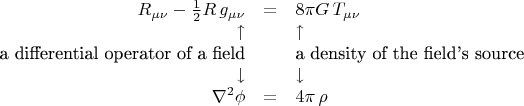$\begin{array}{rcl} R_{\mu\nu}-\tfrac{1}{2}R\,g_{\mu\nu}& = & 8\pi G\, T_{\mu\nu} \\ \uparrow & & \uparrow \\ \text{a differential operator of a field}& & \text{a density of the field's source} \\ \downarrow & & \downarrow \\ \nabla^2\phi & = & 4\pi\, \rho \end{array}$So, the equation does not say anything about geometry of the Einstein tensor. It just says that this tensor, whatever it is, is equal to the content of matter in the same point of space-time.And then, I advice you another book: The Emperor's New Mind, by Penrose, the GR being described in chapter 5. There are vivid explanations of what Riemann, Weyl and Ricci tensors are, and what the Einstein's equation is, with helpful figures. Also, in more depth, they are explained in his The Road to Reality, chapters 17 and 19.Re: Риччи Тензор24/07/12
25
 Well, Poisson's equation is a weak field limit of Einstein Field Equations; so it is only a special case when gravity is weak. And furthermore, Poisson's equation contains second derivatives of the Newtonian gravitational potential. Basically, it says that how gravitational potential varies all over the place is some constant (4piG) times the density? Look, here I have the same kind of confusion. I have Hartle's Intro in GR yet it's a 500+ page book to read and I am too busy to spend so much time on that. What I need is a clear concise explanation.Re: Риччи Тензор
 Заслуженный участник30/01/06
02/09/19
70490
 Последний раз редактировалось Munin 23.12.2013, 18:35, всего редактировалось 2 раз(а). GRstudy в сообщении #598849 писал(а):Parallel transport along a closed loop is a test of presence of curvature which is created by deficit angle. That is only when you consider a 2-dimensional manifold. When you have more dimensions, you can test what will become a vector that is initially pointing perpendicular to the loop's tangent plane. And it can also be skewed some way in the end. Maybe in some fourth orthogonal direction, if this is allowed by the dimension nubmer.GRstudy в сообщении #598849 писал(а):Riemann tensor has 4 indexes: 2 lower have to do with a plane; upper with a vector that we are transporting; last one with a tangent vector, I guess. Last one shows where the initial vector will be skewed to after a trip.GRstudy в сообщении #598849 писал(а):On the contrary, Ricci Tensor is a more complicated story. An idea, how can volume deviate is very unclear.Ok, look at some curved 2-dimensional surface. You can not only transport vectors along it, you can also measure areas. And if you take the Gaussian curvature, you will find that these things are related to each other. Namely, you can mark a small 'cap' on the surface, selecting some center point, and stepping some fixed distance from it in every direction. Then you can calculate two measures of curvature:1. ratio of the circumference of the 'cap' to its radius;2. ratio of the area of the 'cap' to the square of its raduis.Sure, both numbers won't be equal to$2\pi$ and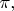$\pi,$ and sure, they will approach those values in a limit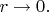$r\to 0.$ But the difference to a limit value, divided by an appropriate power of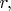$r,$ will approach to some fixed non-zero number, and this number will be the Gaussian curvature, in both cases. This means, whatever is done to the surface in terms of lengths, the same happens in terms of surface measure. When you raise dimensions, you will talk about volume intead of area, but the idea will be the same.-- 25.07.2012 01:53:23 --GRstudy в сообщении #598939 писал(а):Basically, it says that how gravitational potential varies all over the place is some constant (4piG) times the density? Look, here I have the same kind of confusion.Ok, we should go back to the idea of a field source. There are many field equations that have structure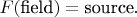$F(\text{field})=\text{source}.$ Maybe it's early to study GR if you are not familiar with other examples of (physical) fields. The Poisson Equation is an archetype case. Now remember how it works in electrostatics: there are some 'field lines' represented mathematically as a vector field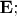$\mathbf{E};$ the lines are related to the potential by$\nabla\phi=-\mathbf{E},$ and to the charges by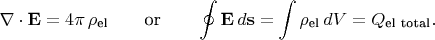$\nabla\cdot\mathbf{E}=4\pi\,\rho_{\text{el}}\qquad\text{or}\qquad\oint\mathbf{E}\,d\mathbf{s}=\int\rho_{\text{el}}\,dV=Q_{\text{el total}}.$ The last equation is most important: it says that lines never break and never end, unless thes come from some charge, or go into some negative charge. By analogy to the flow of fluid, these points, where lines 'flow out' or 'flow in', are called the sources and drains of the vector field. Or shortly, just sources (positive or negative).When you established the picture with sources and vector field lines, we can think of the potential again. Imagine some 2-dimensional plane, then the scalar potential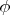$\phi$ could be viewed as some 'lanscape' over it - a surface specified by the function$\phi(x,y).$ On this surface there can be slopes and plains, hills and cavities, mountains and mountain passes. All these features somehow correspond to the picture of lines. What we are interested in are second derivatives - features that look like humps and hollows when dissected by a vertical plane. There are three kinds of those: a hill, a cavity, a saddle. Now, when we are in space between charges, the second derivative of$\phi$ is zero, so the landscape can have a shape only of plains, slopes and saddles. And when we are inside some charged space, say in the uniformly charged ball, the shape of the landscape can be a hill (if the charge density is positive) or a cavity (if negavive).So that is what the source is in the thery of fields: it is the point where something external influences the field, something interacts with it, and then the field cannot be uniform in that point. If the equation refers to the heat transport, then sources are where the heat is extracted. If the equation refers to the wave propagation, then sources are points (or regions) of wave radiation. And so on. Regarding gravitation, the sources should be attracting masses, which make the field be pulling any test body in their direction. In the (gravitational now) Poisson's equation, attracting masses make cavities in the landscape, and around these cavities there should be slopes, because the lanscape cannot immediately become flat again without violation of the Poisson's Equation. And those slopes make test bodies roll down, towards the attracting masses, exactly what we wanted out mathematical model to show.Re: Риччи Тензор02/09/11
1247
Энск
 Последний раз редактировалось zask 25.07.2012, 07:44, всего редактировалось 1 раз. С чего народ перешел на английский? По-моему г-н Зубелевич просто попытался пошутить. Похоже, попытка не удалась...Re: Риччи Тензор24/07/12
25
 Последний раз редактировалось GRstudy 25.07.2012, 09:56, всего редактировалось 1 раз. Thanks for reply!I have followed your instructions and I put them into a visual representation below:Uploaded with ImageShack.usThe circle lies in the plane (NOT tangent to the plane!). Obviously, as you mentioned, 2 pi and pi won't be correct ratios because the surface is curved. This idea, makes volume deviation more clear. Basically, Ricci tensor is something that shows how volume of ball in curved spaces differs from that in flat spaces. You made a perfect job at explaining how volume can deviate. --------------------------------------------------------------------About Riemann tensor: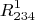$R^{1}_{234}$1-change in a vector which was transported around a closed loop3-4--the plane 2-initial vector--------------------------------------------------------------------Basically, the divergence, the measure how the field spreads out, of a gravitational acceleration, g, is - 4 pi G rho.$\nabla g = - 4 \pi G \rho$, where both g and rho are functions of x,y,z---------------------------------------------------------------------я забыл сказать что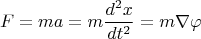$F=ma=m\frac{d^2x}{dt^2}=m\nabla \varphi$Показать сообщения за: Все сообщения1 день7 дней2 недели1 месяц3 месяца6 месяцев1 год Поле сортировки АвторВремя размещенияЗаголовок по возрастаниюпо убыванию

Модераторы: Jnrty, whiterussian, profrotter, Парджеттер, Eule_A, Pphantom, photon, Aer, Супермодераторы

#### Кто сейчас на конференции

Сейчас этот форум просматривают: нет зарегистрированных пользователей

 Вы не можете начинать темыВы не можете отвечать на сообщенияВы не можете редактировать свои сообщенияВы не можете удалять свои сообщенияВы не можете добавлять вложения

 Найти:
Powered by phpBB © 2000, 2002, 2005, 2007 phpBB Group